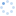# error C2664: “std::list<_Ty>::list(const std::allocator<_Ty> &)”: 不能将参数 1 从 “std::vector<_Ty>”转换为“const std::allocator<_Ty> &”

``````error C2664: “std::list<_Ty>::list(const std::allocator<_Ty> &)”: 不能将参数 1 从
“std::vector<_Ty>”转换为“const std::allocator<_Ty> &”
with
[
_Ty=int
]
原因如下: 无法从“std::vector<_Ty>”转换为“const std::allocator<_Ty>”
with
[
_Ty=int
]
没有可用于执行该转换的用户定义的转换运算符，或者无法调用该运算符
prog33.cpp(13) : error C2664: “std::vector<_Ty>::vector(const std::allocator<_Ty> &)”: 不能将参数
1 从“std::vector<_Ty>”转换为“const std::allocator<_Ty> &”
with
[
_Ty=double
]
and
[
_Ty=int
]
and
[
_Ty=double
]
原因如下: 无法从“std::vector<_Ty>”转换为“const std::allocator<_Ty>”
with
[
_Ty=int
]
and
[
_Ty=double
]
没有可用于执行该转换的用户定义的转换运算符，或者无法调用该运算符``````

``````
#include <iostream>
#include <vector>
#include <list>
using std::vector;
using std::list;
using std::cout;
//容器初始化举例
int main()
{
vector<int>	ivec;//使用默认构造函数
vector<int>	ivec2(ivec);//初始化为同型容器的副本
list<int>	ilist(ivec);//错误，容器类型不同
vector<double>	dvec(ivec);//错误，容器元素类型不同

return 0;
}``````

``````vector<int>    ivec;

list<int>   ilist(ivec.begin(),ivec.end());
vector<double>    dvec(ivec.begin(),ivec.end());``````评论加载中，请稍后...